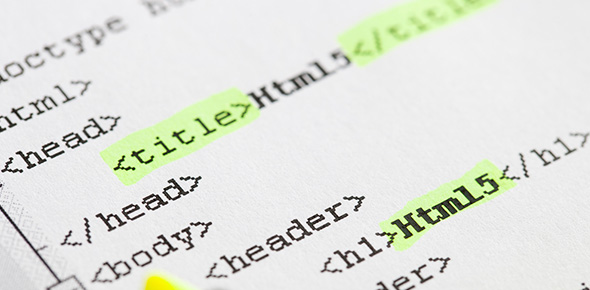# Test Your Basic PHP Knowledge

10 Questions | Attempts: 219SettingsThis is a quiz that tests your basic PHP.

• 1.
What is output ? echo "asdf"; // prints asdf ?> echo "qwer"; ?>
• A.

Asdfqwer

• B.

Asdf qwer

• C.

Asdf echo "1234";

• 2.
How amny scalar types are there in PHP ?
• A.

4

• B.

6

• C.

8

• D.

10

• 3.
What is output of following code?     \$i=(bool)0.0;   if(\$i) { echo "in if"; } else { echo "in else"; }   ?>
• A.

In if

• B.

In else

• 4.
<?php   \$i=(bool) "0";   if(\$i) { echo "in else"; } else { echo "in if"; }   ?>
• A.

In if

• B.

In else

• 5.
<?php   \$i=(bool) array();   if(\$i) { echo "in if"; } else { echo "in else"; }   ?>
• A.

In if

• B.

In else

• 6.
<?php   \$i=(bool) array(1 => "false");   if(\$i) { echo "in if"; } else { echo "in else"; }   ?>
• A.

In if

• B.

In else

• 7.
<?php   \$i=01291;   echo \$i;   ?>
• A.

01291

• B.

012

• C.

10

• D.

15

• 8.
How many types of strings are there in PHP ?
• A.

2

• B.

3

• C.

4

• 9.
\$i="asdf";     echo \$i["3"];
• A.

Syntax error

• B.

D

• C.

F

• 10.
\$i =(int)"123asdf"; \$i++; echo \$i;
• A.

Error

• B.

123

• C.

124

## Related TopicsBack to top
×

Wait!
Here's an interesting quiz for you.